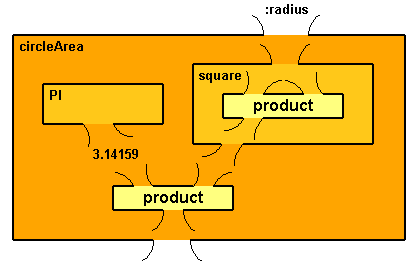BFOIT - Introduction to Computer Programming

## Lastly

1. In the previous lesson you wrote printCircleArea.  Write a procedure named circleArea with an input for the radius of the circle.  It should produce an output that is the area of the circle.  Test it with the inputs in Table 9.1 and make sure you get good answers.

Here is the source code.  Note that the only difference between this code and printCircleArea is changing the println command to an output command.

 `````` to circleArea :radius output product 3.1416 (product :radius :radius)    end ``````
2. Part of the arithmetic expression you wrote to compute the area of a circle involved squaring a number.  Squaring a number is a common thing to do.  Write a procedure named square with an input :number that produces number-squared.  There is also a constant value in the expression, PI, which should be defined as a symbolic constant - define the procedure PI which outputs 3.14159.  Redefine your circleArea using these new procedures.  Once again, test it...

Here is an enhanced plumbing diagram for a solution.What is different with this plumbing diagram is that I've added boxes for user-defined operators.  The built-in operators (product) are colored light yellow, like in all of the plumbing diagrams you've seen.  What is new is the boxes representing the operators you were to define.  The names of these operators are in the upper-left corner of the boxes instead of being in the center.  They have optional inputs and outputs like those you have seen with the built-in operators.

Look at the box representing the circleArea operator.  It has a single input (:radius) on its top edge.  Inside the box are three operators; two other operators you were to define (PI and square) and one built-in operator (product).

Since PI and square are user-defined operators they are represented as boxes with their names in the top-left corner.  PI has no input; it simply outputs the number 3.14159.  square has one input which is fed into both of the inputs to product.  Its output becomes the output of square.

Finally, the outputs from PI and square are fed into circleArea's product operator.  Its output becomes the output of circleArea.

And here is the source code for this diagram.

 `````` ; Symbolic Constant Pi ; the ratio of the circumference of a circle to its diameter    to PI output 3.14159 end ; Output a number multiplied by itself to square :number output product :number :number end ; Output the area of a circle given its radius to circleArea :radius output product PI (square :radius) end ``````